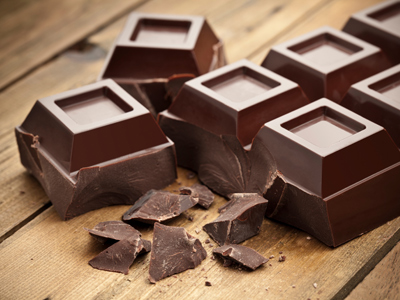What would be a suitable unit to measure the area of a chunk of chocolate?

# Perimeter and Area (Year 5)

In KS2 Maths children will come to understand perimeter and area. In Year Five children should know how to work out the perimeter of squares and rectangles and will now be introduced to other regular shapes like a pentagon or a hexagon and shown how to calculate its area. They should be confident when working out the area of squares and rectangles and they will also be expected to know that square metres contain 10,000 square cm and that square cm contain 100 square mm.

The perimeter of a shape is the distance around its edge. To work out the perimeter of a hexagon multiply the length of one of its sides by 6. If its sides are 2 metres long its perimeter would be 12 metres. The area is the size of the surface. Knowing the area of a wall is important if you are planning to give it a lick of paint - otherwise how will you know how many tins of paint to buy?!

Can you work out the perimeter of a hexagon? Find out what you know about area and perimeter by taking this quiz for 9-10 year olds.

1.

Which would be best measured in cm2?

Area of a playground
Area of a town
Area of a desk top
Area of a pen tip
Towns might be measured in km2, playgrounds in m2 and pen tips in mm2
2.
What is the formula for calculating the area of a rectangle?
2 x length x 2 x width
2 x length + 2 x width
Length + width
Length x width
If a rectangle measured 7cm by 10cm its area would be 70cm2
3.
How many cm2 is equivalent to 1m2?
10cm2
100cm2
1,000cm2
10,000cm2
There are 100cm in 1m so 100 x 100 = 10,000
4.
What is the formula for calculating the perimeter of a rectangle?
2 x length x 2 x width
2 x length + 2 x width
Length + width
Length x width
2 sides of a rectangle are one length whilst the other two sides are a different length, for example 2 sides are 4cm long and the other 2 sides are 6cm long. This rectangle would have a perimeter of 20cm
5.
What is the perimeter of a regular pentagon with sides of 5cm?
24cm
100cm
10cm
25cm
A pentagon has 5 sides, therefore 5 x 5cm will give you the perimeter
6.
A rectangle has a perimeter of 38cm. The shortest sides are 7cm. What length are the two longest sides?
12cm
14cm
21cm
28cm
2 x 7 = 14
38 - 14 = 24
24 ÷ 2 = 12
7.
Which would be a good estimate of the area of a classroom?
1,000mm2
100mm2
10m2
100m2
10m2 would be large enough for a room in a house but not big enough for a classroom
8.
A regular hexagon has a perimeter of 54cm. What is the length of the sides?
6cm
9cm
12cm
20cm
A regular hexagon has 6 equal sides. To calculate the length of each side, divide the perimeter by 6
9.
Which would be a suitable unit to measure the area of a chunk of chocolate?
Square grams
Square metres
Square millimetres
Square millilitres
Grams measure weight and millilitres measure volume. Square metres measure area but chocolate bars aren't that big!
10.
What is the formula for calculating the perimeter of a regular hexagon?
4 x length of side
6 x length of side
6 x length + width
Length of side divided by 6
As a regular hexagon has 6 equal sides, just multiply the length of the sides by 6